# SAT Math Multiple Choice Question 961: Answer and Explanation

### Test Information

Question: 961

13.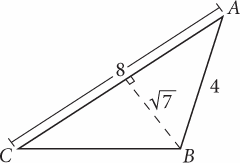Note: Figure not drawn to scale.

In the figure above, what is the length of side BC?

• A.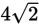• B.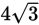• C.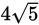• D.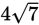Explanation:

A

Difficulty: Hard

Category: Additional Topics in Math / Geometry

Strategic Advice: Work with right triangles, whenever possible, so you can use properties and theorems that allow you to find side lengths.

Getting to the Answer: Add a point (call it D) to the diagram where the dashed line meets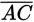, as shown below: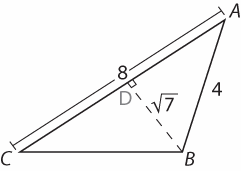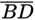breaks ΔABC into two smaller right triangles. You know two side lengths for ΔABD, so use the Pythagorean theorem to find the third side (which happens to be a leg of the triangle):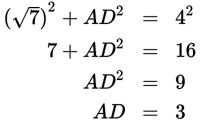Now,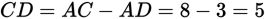. Use the Pythag-orean theorem again to find BC, keeping in mind that BC is the hypotenuse this time: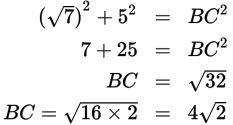Choice (A) is correct.